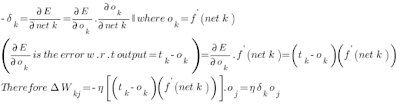# Assigning Membership Values using Artificial Neural Networks

### Characteristics of Artificial Neural Networks (ANN)

1. ANN’s are biologically inspired.
2. ANN’s are organized in a way that may or may not be related to the anatomy of the brain.
3. They learn from past experiences.
4. A neuron was designed to imitate the first order characteristics of a biological neuron.
5. ANN’s resemble brain in two aspects, one of which is that their knowledge is acquired by the network through a learning process and the other is, inter-neuron connects weights known as synaptic weights which are used to store the knowledge.

### Basic Features of ANN :-

1. High computational rates due to massive parallel processing.
2. Fault tolerance is high as damage to few nodes doesn’t significantly effect the overall performance.
3. With Learning and Training feature the network adopts itself based on the information received by it in the past environment.
4. ANN’s feature Goal Seeking where the performance to achieve the goal is measured and is used to self organize the system.
5. They are Primitive Computational Elements as each element resembles one simple logical neuron and it cannot do much.
6. #### Back Propagation Algorithm

ni be number of nodes in the input layer.
nj be number of nodes in the hidden layer.
nk be number of nodes in the output layer.

here hidden and output layers are modeled and input layer takes the inputs only.

#### Forward Pass :-

1. Between i and j layer:-

Here sigmoid function is taken as non-linear function

2. Between j and k layer:-

let tk be the target at the kth node.
Evaluate mean sum square error

where,

• P represents particular pattern.
• k represents number of nodes of output.
• np is number of patterns.

Our aim is to minimize the total error ‘E’, because E should be within the specified limits.

#### Backward pass :-

1. Between output layer and hidden layer :-

Its value is between 0 and 1
–> If η (eta) is too low, rate of convergence is very slow and system will take more time for training.

–> If η is too high, system will be oscillatory.

Now, Define

2. This is the change in weight between output and hidden layers.

3. Between the hidden and input layers :-Thus weights will be adjusted until we get a convergent solution. For different set of patterns we will train this network and then using the remaining patterns we will test it.